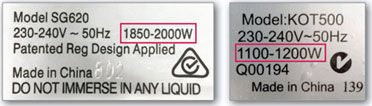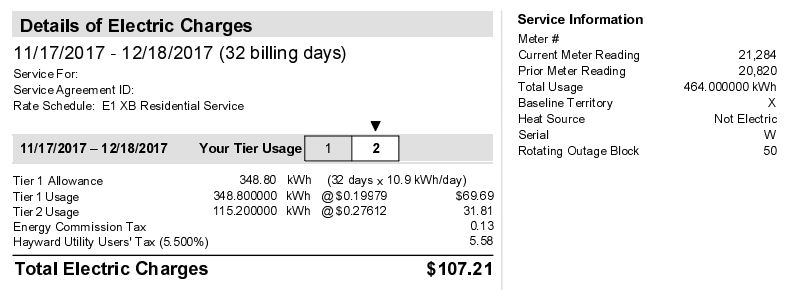# How to Calculate Electric Costs

## Calculate Energy Cost For a Device

1. Read the wattage on the label of the device
If the device doesn’t have a label, you can buy a wattage measuring device, such as the Kill A Watt.2. Calculate Watts Per Day
To calculate energy consumption costs, simply multiply the unit’s wattage by the number of hours you use it to find the number of watt-hours consumed each day. For example, let’s say you use a 125 watt television for three hours per day. By multiplying the wattage by the number of hours used per day, we find that you are using 375 watt-hours per day.125 watts X 3 hours = 375 watt-hours per day
3. Convert to Kilowatts
But electricity is measure in kilowatt hours on your electricity bill. Since we know that 1 kilowatt is equal to 1,000 watts, calculating how many kWh a particular device uses is as easy as dividing by 1,000.375 watt-hours per day / 1000 = 0.375 kWh per day
4. Calculate Usage Over a Month Period
Now to find out how much that’s actually going to cost you on your electric bill, you’ll have to take the equation a bit further. First you’ll need to figure out how many kWh the TV uses per month.375 watt-hours per day X 30 days = 11.25 kWh per month
5. Calculate Monthly Cost
Next, pull out your last electric bill and see how much you pay per kWh. For this example, let’s say you pay 10 cents per kilowatt hour. To find how much the TV is costing you in a month, multiply your electricity rate by the kWh per month that you calculated above.
11.25 kWh per month X \$0.10 per kWh = \$1.13 per month

## Another Example

Here’s another more costly example: Your refrigerator runs 24 hours a day. Most refrigerators consume 300-780 watts of electricity. Let’s assume you bought a model that uses only 300 watts.

300 watts X 24 hours = 7,200 watt-hours per day
7,200 watt-hours per day / 1000 = 7.2 kWh per day
7.2 kWh per day X 30 days = 216 kWh per month
216 kWh per month x \$0.10 per kWh = \$21.60 per month

## Electric Bill

In the electric bill below, we see that pricing is based on tiers.

• Tier 1 costs about \$0.20 per kWh with a monthly allowance of 348.8 kWh. Monthly usage over 348.8 kWh will be charged Tier 2 pricing.
• Tier 2 costs about \$0.28 per kWh.

The total usage for the month was 464 kWh. The calculations in the bill show how the costs break down.## Common Wattages for Household Appliances

The wattage on appliances or electronics varies by device. Typically, older model appliances use more energy, but newer models tend to be more efficient. You can also purchase ENERGY STAR appliances, which are among the most efficient appliances. According to the EPA, here’s a list of typical wattage levels for your everyday devices.

 Coffee maker 900-1200 watts Microwave 750-1100 watts Toaster 800-1400 watts Dishwasher 1200-2400 watts Washer 350-500 watts Dryer 1800-5000 watts Iron 100-1800 watts Ceiling fan 65-175 watts Space heater (40gal) 4500-5500 watts Hair dryer 1200-1875 watts Laptop 50 watts Computer monitor 150 watts Computer tower 120 watts Television 19″-36″ 65-133 watts Television 53″-61″ 170 watts